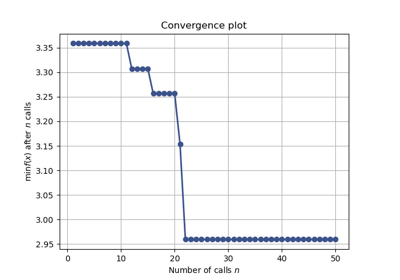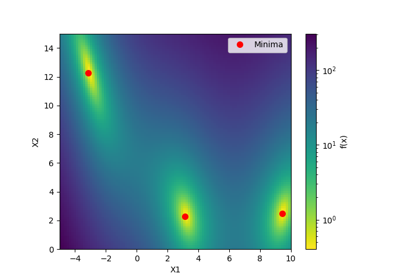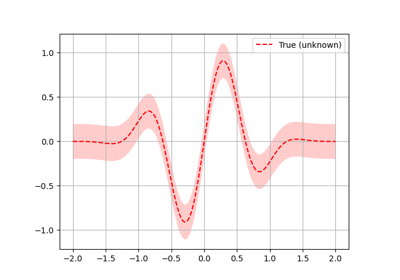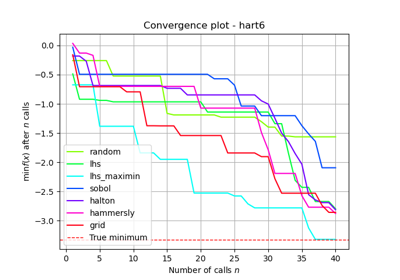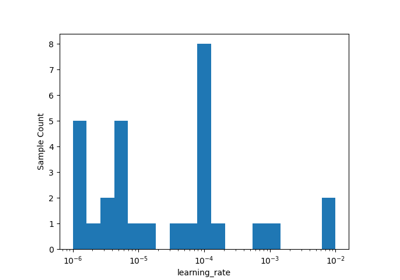# skopt.gp_minimize¶

skopt.gp_minimize(func, dimensions, base_estimator=None, n_calls=100, n_random_starts=None, n_initial_points=10, initial_point_generator='random', acq_func='gp_hedge', acq_optimizer='auto', x0=None, y0=None, random_state=None, verbose=False, callback=None, n_points=10000, n_restarts_optimizer=5, xi=0.01, kappa=1.96, noise='gaussian', n_jobs=1, model_queue_size=None)[source][source]

Bayesian optimization using Gaussian Processes.

If every function evaluation is expensive, for instance when the parameters are the hyperparameters of a neural network and the function evaluation is the mean cross-validation score across ten folds, optimizing the hyperparameters by standard optimization routines would take for ever!

The idea is to approximate the function using a Gaussian process. In other words the function values are assumed to follow a multivariate gaussian. The covariance of the function values are given by a GP kernel between the parameters. Then a smart choice to choose the next parameter to evaluate can be made by the acquisition function over the Gaussian prior which is much quicker to evaluate.

The total number of evaluations, n_calls, are performed like the following. If x0 is provided but not y0, then the elements of x0 are first evaluated, followed by n_initial_points evaluations. Finally, n_calls - len(x0) - n_initial_points evaluations are made guided by the surrogate model. If x0 and y0 are both provided then n_initial_points evaluations are first made then n_calls - n_initial_points subsequent evaluations are made guided by the surrogate model.

The first n_initial_points are generated by the initial_point_generator.

Parameters
funccallable

Function to minimize. Should take a single list of parameters and return the objective value.

If you have a search-space where all dimensions have names, then you can use skopt.utils.use_named_args() as a decorator on your objective function, in order to call it directly with the named arguments. See use_named_args for an example.

dimensions[list, shape (n_dims,)

List of search space dimensions. Each search dimension can be defined either as

• a (lower_bound, upper_bound) tuple (for Real or Integer dimensions),

• a (lower_bound, upper_bound, "prior") tuple (for Real dimensions),

• as a list of categories (for Categorical dimensions), or

• an instance of a Dimension object (Real, Integer or Categorical).

Note

The upper and lower bounds are inclusive for Integer dimensions.

base_estimatora Gaussian process estimator

The Gaussian process estimator to use for optimization. By default, a Matern kernel is used with the following hyperparameters tuned.

• All the length scales of the Matern kernel.

• The covariance amplitude that each element is multiplied with.

• Noise that is added to the matern kernel. The noise is assumed to be iid gaussian.

n_callsint, default: 100

Number of calls to func.

n_random_startsint, default: None

Number of evaluations of func with random points before approximating it with base_estimator.

Deprecated since version 0.8: use n_initial_points instead.

n_initial_pointsint, default: 10

Number of evaluations of func with initialization points before approximating it with base_estimator. Initial point generator can be changed by setting initial_point_generator.

initial_point_generatorstr, InitialPointGenerator instance, default: ‘random’

Sets a initial points generator. Can be either

• "random" for uniform random numbers,

• "sobol" for a Sobol sequence,

• "halton" for a Halton sequence,

• "hammersly" for a Hammersly sequence,

• "lhs" for a latin hypercube sequence,

acq_funcstring, default: "gp_hedge"

Function to minimize over the gaussian prior. Can be either

• "LCB" for lower confidence bound.

• "EI" for negative expected improvement.

• "PI" for negative probability of improvement.

• "gp_hedge" Probabilistically choose one of the above three acquisition functions at every iteration. The weightage given to these gains can be set by $$\eta$$ through acq_func_kwargs.

• The gains g_i are initialized to zero.

• At every iteration,

• Each acquisition function is optimised independently to propose an candidate point X_i.

• Out of all these candidate points, the next point X_best is chosen by $$softmax(\eta g_i)$$

• After fitting the surrogate model with (X_best, y_best), the gains are updated such that $$g_i -= \mu(X_i)$$

• "EIps" for negated expected improvement per second to take into account the function compute time. Then, the objective function is assumed to return two values, the first being the objective value and the second being the time taken in seconds.

• "PIps" for negated probability of improvement per second. The return type of the objective function is assumed to be similar to that of "EIps"

acq_optimizerstring, "sampling" or "lbfgs", default: "lbfgs"

Method to minimize the acquisition function. The fit model is updated with the optimal value obtained by optimizing acq_func with acq_optimizer.

The acq_func is computed at n_points sampled randomly.

• If set to "auto", then acq_optimizer is configured on the basis of the space searched over. If the space is Categorical then this is set to be "sampling".

• If set to "sampling", then the point among these n_points where the acq_func is minimum is the next candidate minimum.

• If set to "lbfgs", then

• The n_restarts_optimizer no. of points which the acquisition function is least are taken as start points.

• "lbfgs" is run for 20 iterations with these points as initial points to find local minima.

• The optimal of these local minima is used to update the prior.

x0list, list of lists or None

Initial input points.

• If it is a list of lists, use it as a list of input points.

• If it is a list, use it as a single initial input point.

• If it is None, no initial input points are used.

y0list, scalar or None

Evaluation of initial input points.

• If it is a list, then it corresponds to evaluations of the function at each element of x0 : the i-th element of y0 corresponds to the function evaluated at the i-th element of x0.

• If it is a scalar, then it corresponds to the evaluation of the function at x0.

• If it is None and x0 is provided, then the function is evaluated at each element of x0.

random_stateint, RandomState instance, or None (default)

Set random state to something other than None for reproducible results.

verboseboolean, default: False

Control the verbosity. It is advised to set the verbosity to True for long optimization runs.

callbackcallable, list of callables, optional

If callable then callback(res) is called after each call to func. If list of callables, then each callable in the list is called.

n_pointsint, default: 10000

Number of points to sample to determine the next “best” point. Useless if acq_optimizer is set to "lbfgs".

n_restarts_optimizerint, default: 5

The number of restarts of the optimizer when acq_optimizer is "lbfgs".

kappafloat, default: 1.96

Controls how much of the variance in the predicted values should be taken into account. If set to be very high, then we are favouring exploration over exploitation and vice versa. Used when the acquisition is "LCB".

xifloat, default: 0.01

Controls how much improvement one wants over the previous best values. Used when the acquisition is either "EI" or "PI".

noisefloat, default: “gaussian”
• Use noise=”gaussian” if the objective returns noisy observations. The noise of each observation is assumed to be iid with mean zero and a fixed variance.

• If the variance is known before-hand, this can be set directly to the variance of the noise.

• Set this to a value close to zero (1e-10) if the function is noise-free. Setting to zero might cause stability issues.

n_jobsint, default: 1

Number of cores to run in parallel while running the lbfgs optimizations over the acquisition function. Valid only when acq_optimizer is set to "lbfgs". Defaults to 1 core. If n_jobs=-1, then number of jobs is set to number of cores.

model_queue_sizeint or None, default: None

Keeps list of models only as long as the argument given. In the case of None, the list has no capped length.

Returns
resOptimizeResult, scipy object

The optimization result returned as a OptimizeResult object. Important attributes are:

• x [list]: location of the minimum.

• fun [float]: function value at the minimum.

• models: surrogate models used for each iteration.

• x_iters [list of lists]: location of function evaluation for each iteration.

• func_vals [array]: function value for each iteration.

• space [Space]: the optimization space.

• specs [dict]: the call specifications.

• rng [RandomState instance]: State of the random state at the end of minimization.

For more details related to the OptimizeResult object, refer http://docs.scipy.org/doc/scipy/reference/generated/scipy.optimize.OptimizeResult.html

## Examples using skopt.gp_minimize`¶